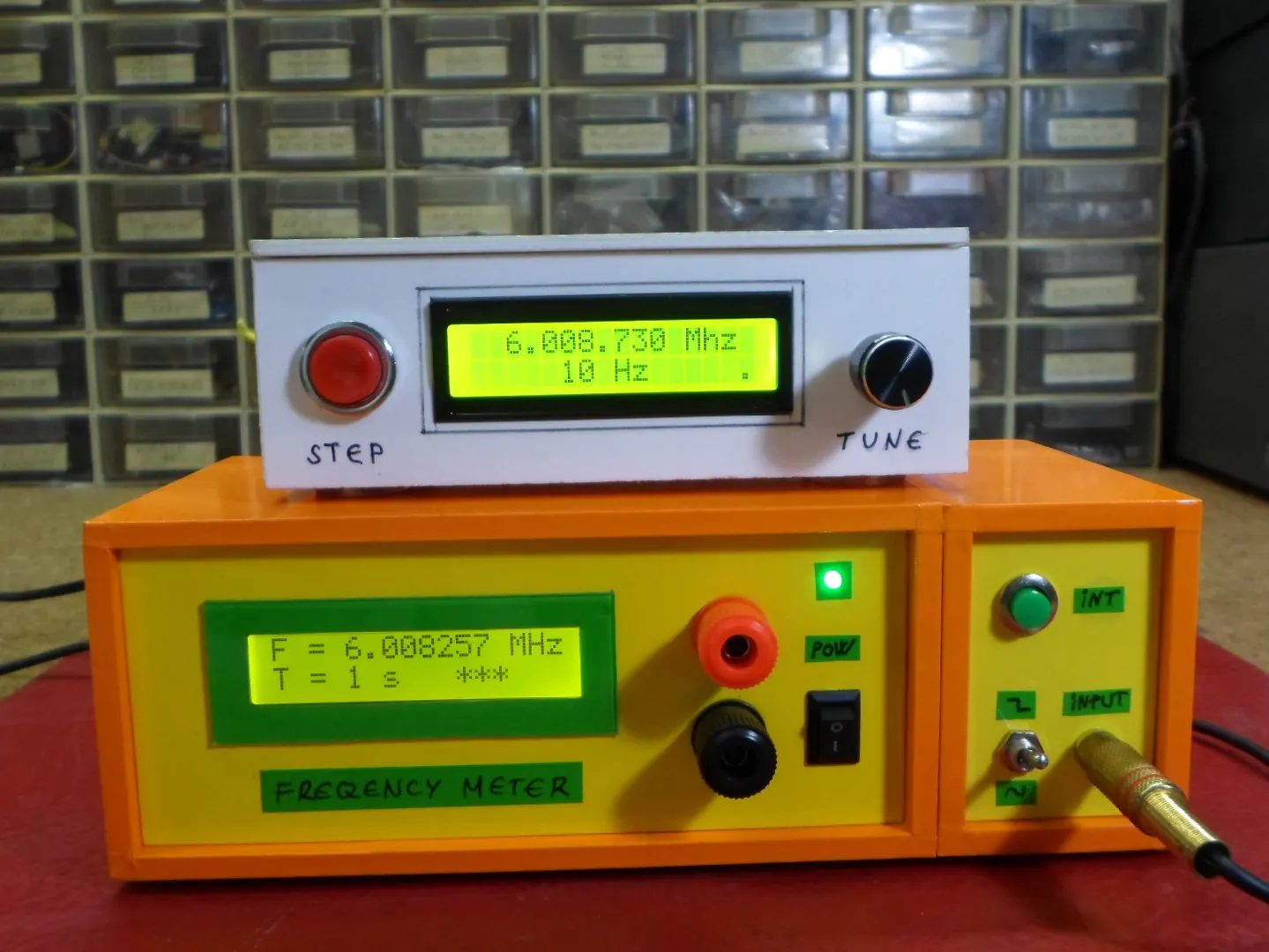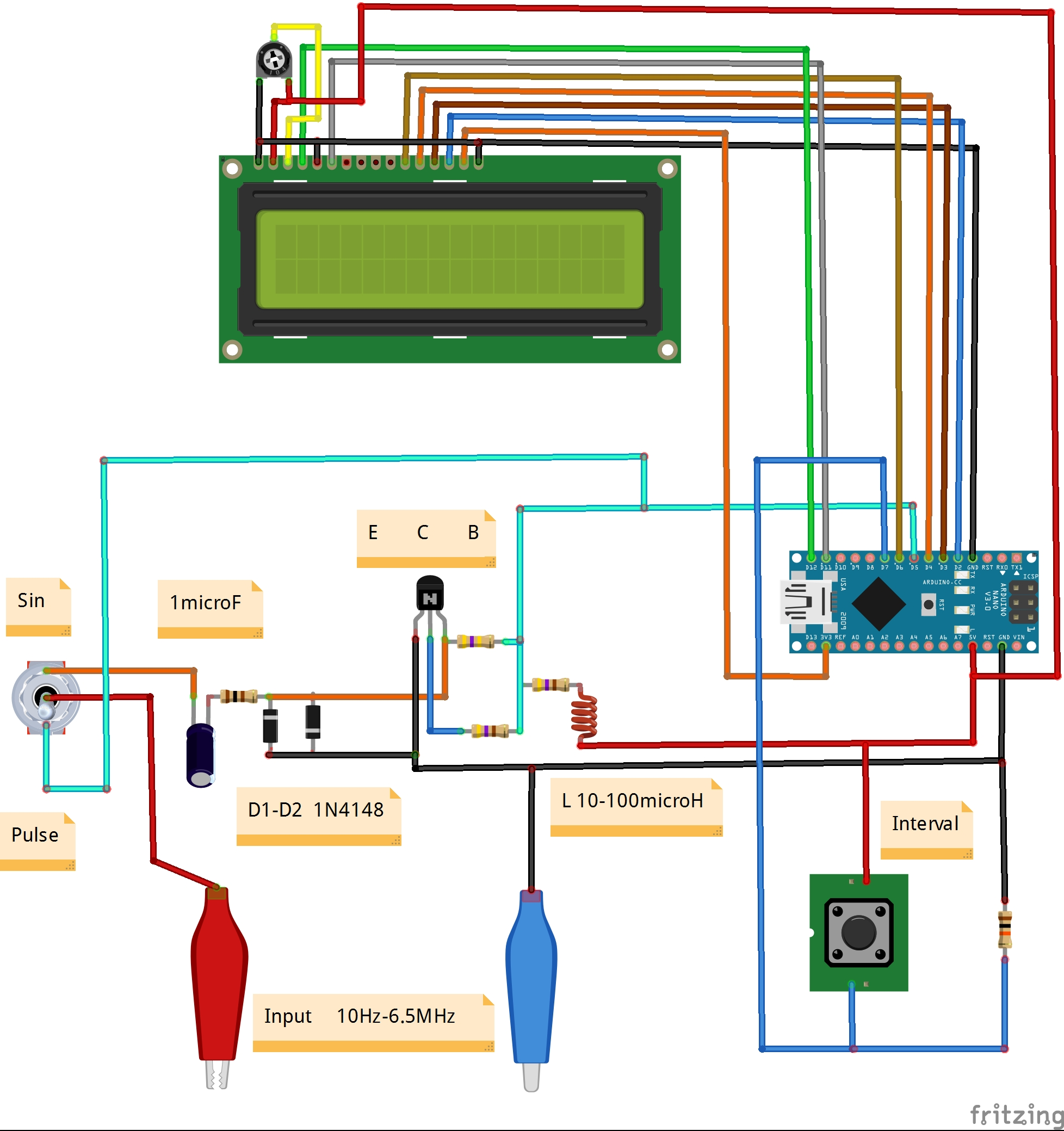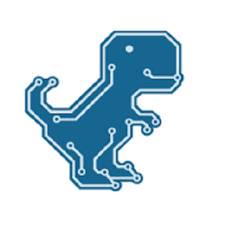Project tutorial# DIY Simple Frequency Meter Up to 6.5MHz © GPL3+

Another simple to build useful instrument in you lab.

• 228 views
• 1 respect

## Necessary tools and machinesSoldering iron (generic)

## Apps and online services

The device presented in the video is a frequency meter made using an Arduino Nano microcontroller. It can measure the frequency of signals with rectangular, sinusoidal and triangular shapes.

Its measurement range is from a few hertz to 6.5 Megahertz. Three measurement time intervals are also available - 0.1, 1 and 10 seconds. If we measure only rectangular signals, then there is no need for a shaping amplifier and the signal is fed directly to the digital pin 5 from Arduino.

The code is very simple thanks to the "FreqCount" library which you can also download below.

The device is very simple and consists of several components:

- Arduino Nano microcontroller

- Shaping amplifier board

- LCD display

- Input signal shape selector

- Input JACK

- and Time interval switch : we can choose three intervals 0.1 -1 -and 10 seconds.

As you can see in the video, the instrument is very precise in the whole range, and we can also calibrate the frequency meter with the simple procedure described below:

In the Arduino libraries folder find the FreqCount library,

in the FreqCount.cpp file find the lines:

#if defined (TIMER_USE_TIMER2) && F_CPU == 12000000L

float correct = count_output * 0.996155;

and replace them with:

#if defined (TIMER_USE_TIMER2) && F_CPU == 16000000L

float correct = count_output * 1.000000;

where 1.000000 is your correction factor, the

correction must be carried out by applying 1 MHz to the input of the frequency meter.

After changing the file, upload a new sketch to the Arduino board.

Finally, the frequency meter is built into a suitable plastic box and is another useful instrument in the electronic laboratory.

## Code

##### CodeC/C++
```#include <FreqCount.h>//https://github.com/PaulStoffregen/FreqCount/archive/master.zip
#include <LiquidCrystal.h>

LiquidCrystal lcd(12, 11, 6, 4, 3, 2);// RS,E,D4,D5,D6,D7
void setup() {
lcd.begin(16, 2);// LCD 16X2
pinMode(7,INPUT);
FreqCount.begin(1000);
}
unsigned long f;float f0;
int x,n=3,r;

void loop() {

lcd.setCursor(0,1);
if(n==1){x++;if(x==1){FreqCount.begin(100);}r=-1;lcd.print("T = 0.1 s ");}
if(n==2){x++;if(x==1){FreqCount.begin(10000);}r=1;lcd.print("T = 10 s ");}
if(n==3){x++;if(x==1){FreqCount.begin(1000);}r=0;lcd.print("T = 1 s  ");}
if(n>3){n=1;}
lcd.setCursor(0,0);
lcd.print("F = ");
if(f>=1000000 && n==3){f0=f/1000000.0;lcd.print(f0,6+r);lcd.print(" MHz");}
if(f<1000000 && n==3){f0=f/1000.0;lcd.print(f0,3+r);lcd.print(" kHz");}
if(f>=100000 && n==1){f0=f/100000.0;lcd.print(f0,6+r);lcd.print(" MHz");}
if(f<100000 && n==1){f0=f/100.0;lcd.print(f0,3+r);lcd.print(" kHz");}
if(f>=10000000 && n==2){f0=f/10000000.0;lcd.print(f0,6+r);lcd.print("MHz");}
if(f<10000000 && n==2){f0=f/10000.0;lcd.print(f0,3+r);lcd.print(" kHz");}

if (FreqCount.available()) {

lcd.setCursor(10,1);lcd.print("***");
}
delay(200);
lcd.clear();
}
```

## Schematics#### Author##### Mirko Pavleski
• 64 projects
• 304 followers

#### Published on

September 15, 2020
See similar projects
you might like

#### DIY Simple Autorange Capacitance Meter (10pF-10000microF)

Project tutorial by Mirko Pavleski

• 2,777 views
• 1 comment
• 9 respects

#### DIY Simple Measuring Wheel with Rotary Encoder

Project tutorial by Mirko Pavleski

• 6,716 views
• 17 respects

#### DIY Si4730 All Band Radio (LW, MW, SW, FM)

Project tutorial by Mirko Pavleski

• 30,156 views
• 63 respects

#### DIY Autorange Ohmmeter

Project tutorial by Mirko Pavleski

• 2,419 views
• 1 comment
• 7 respects

#### DIY Simple Weather Forecast Device

Project tutorial by Mirko Pavleski

• 1,874 views